### Modeling and simulation of convection-dominated species transfer at rising bubbles

First supervisor: Prof. Dr. rer. nat. Dieter Bothe
Second supervisor: Prof. Dr.-Ing. Peter Stephan

## Gas-liquid reactors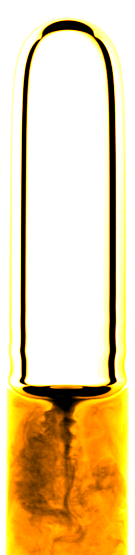micro reactor
size: millimeter
source: SPP 1740

prediction of

• mass transfer
• enhancement
• mixing
• conversion
• selectivity
• yield
• ...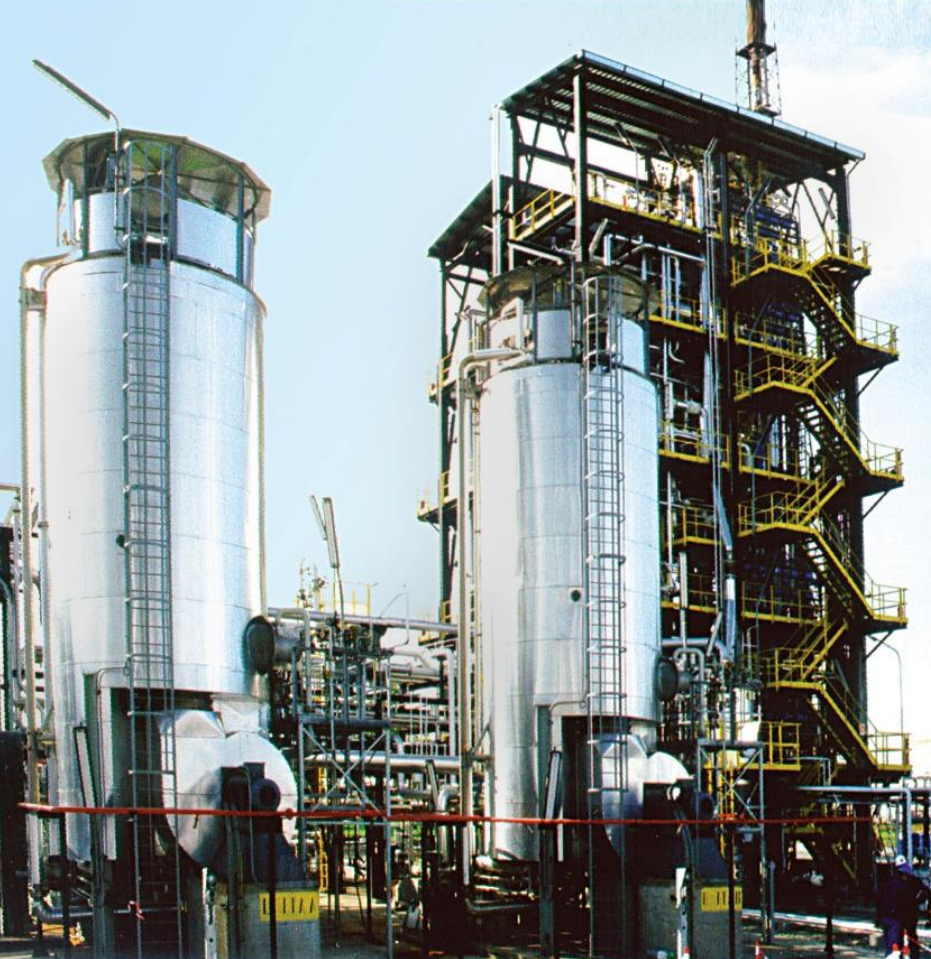bubble column reactor
size: meter
source: R. M. Raimundo, ENI

## High Péclet number problem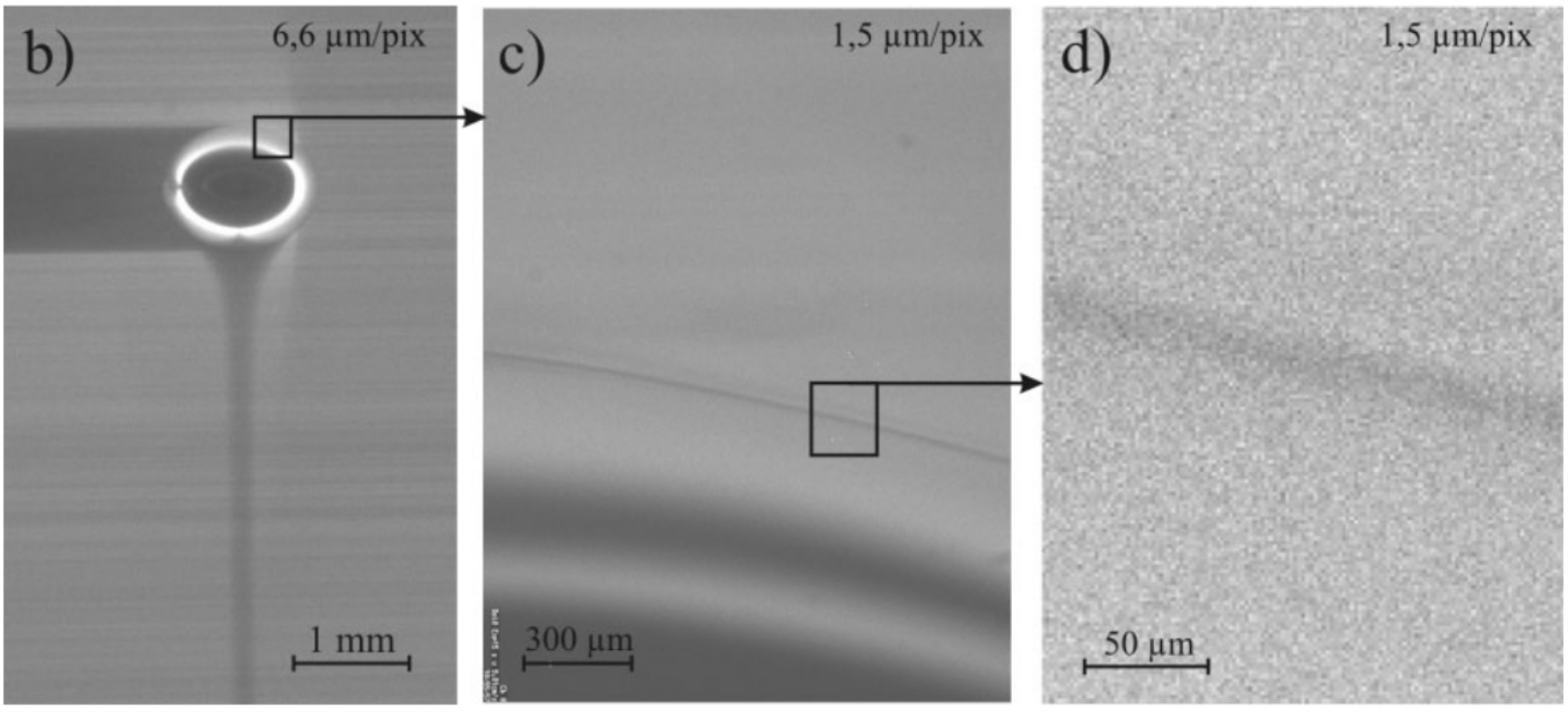## Specimen calculation

$d_b=1~mm$ water/oxygen at room temperature

• $Pe = Sc\ Re = \nu_l / D_{O_2} \cdot U_b d_b/\nu_l \approx 10^5$
• $$Re\approx 250;\quad \delta_h/d_b \propto Re^{-1/2};\quad\delta_h\approx 45~\mu m$$
• $$Sc\approx 500;\quad \delta_c/\delta_h \propto Sc^{-1/2};\quad\delta_c\approx 2.5~\mu m$$

$\delta_h/\delta_c$ typically 10 ... 100

feasible simulations up to $Pe\approx 1000$ (3D, HPC)

## Outline

1. Effect of underresolved meshes
2. Subgrid-scale modeling
3. Data-driven modeling
4. Subgrid-scale model assessment
5. Comparison with experiments
6. Summary and outlook

## Underresolved meshes

$$\partial_t c_A + \nabla \cdot (c_A\mathbf{u} - D_A \nabla c) = 0 \quad \text{in}\quad \Omega^+$$
• $c_A|_\Sigma = const.$
• $\mathbf{u}$ - given velocity vector field
• finite volume discretization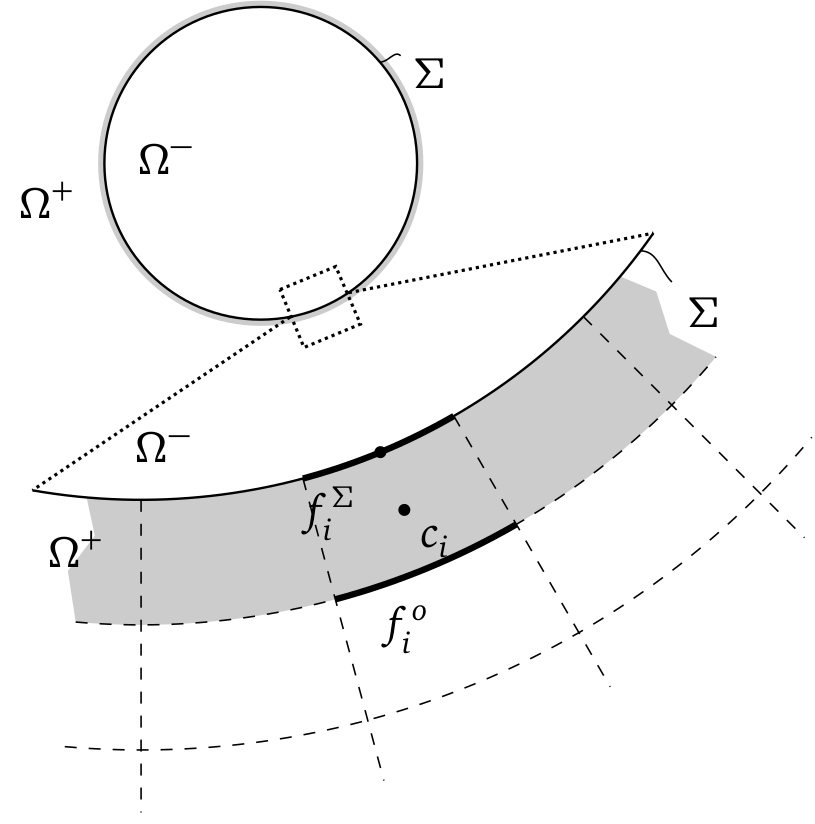$\Omega^\pm$ - liquid/gas domain, $\Sigma$ - interface, $f_i$ - cell faces

## Transfer species - A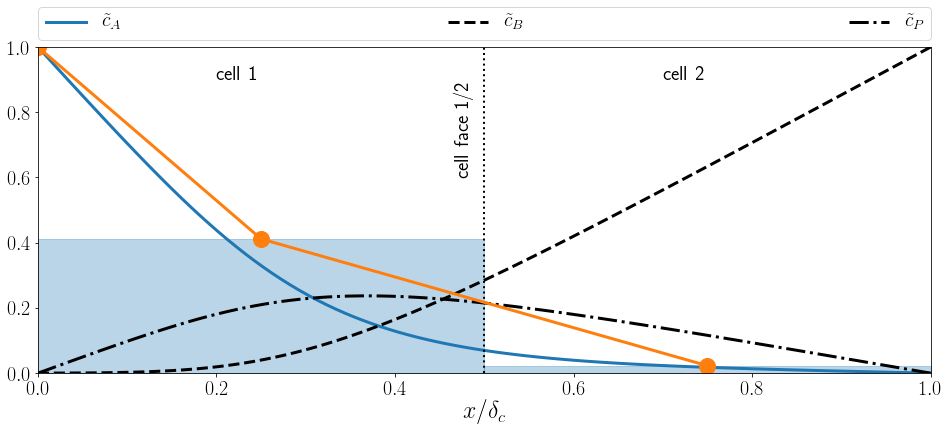Normalized concentration profile $\tilde{c}_A$ in interface normal direction $x/\delta_c$ for the transfer species (solid blue line), average concentration values per cell (shaded blue), and linear reconstruction (orange).

## Subgrid-scale modeling

Idea: Leverage coherent structures in convection-dominated species boundary layers.

1. Substitute problem
Find a representative substitute problem to obtain a parameterized profile function.
2. Reconstruction
Adjust the profile function based on local simulation data (cell-width, average concentration, ...).
3. Correction
Correct convective fluxes, diffusive fluxes, and reaction source terms based on the adjusted profile function.

## (1) Substitute problem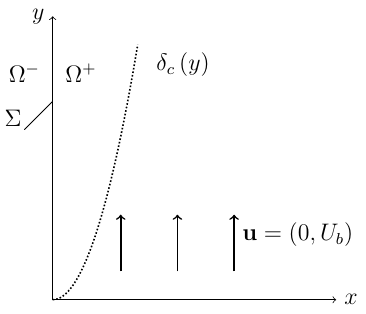dimensionless form
$$\partial_\tilde{y} \tilde{c} = \frac{1}{Pe}\partial_{\tilde{x}\tilde{x}} \tilde{c}$$
• $\tilde{c}(\tilde{x}=0,\tilde{y}) = 1$
• $\tilde{c}(\tilde{x}>0,\tilde{y}=0) = 0$
• $\tilde{c}(\tilde{x}\rightarrow\infty,\tilde{y}) = 0$

## (3.1) Correction

1. compute interface normal derivative:
$\partial_\tilde{x} \tilde{c}|_{\tilde{x}=0} = 2/(\sqrt{\pi}\delta_{num})$
2. correct numerical diffusive flux at $\tilde{x}=0$
3. solve transport equation for $\tilde{c}$

→ saves one refinement level (half cell-width)

D. Bothe, S. Fleckenstein:
A Volume-of-Fluid-based method for mass transfer processes at fluid particles (2013)

## (3.2) Correction

Idea:
Correct convective and diffusive fluxes over all cell faces of an interface cell.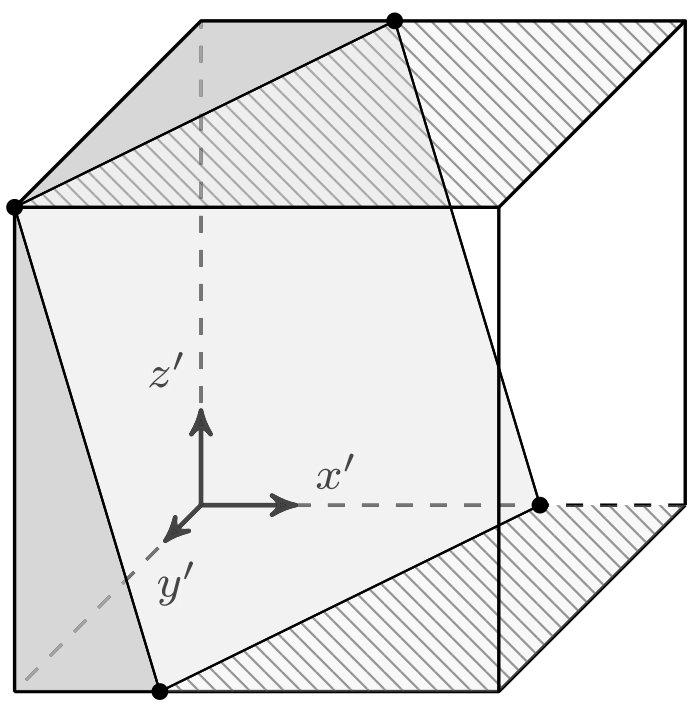Control volume (cube) with embedded interface (plane).
A. Weiner, D. Bothe: Advanced subgrid-scale modeling for convection-dominated species transport at fluid interfaces with application to mass transfer from rising bubbles (2017)

## Results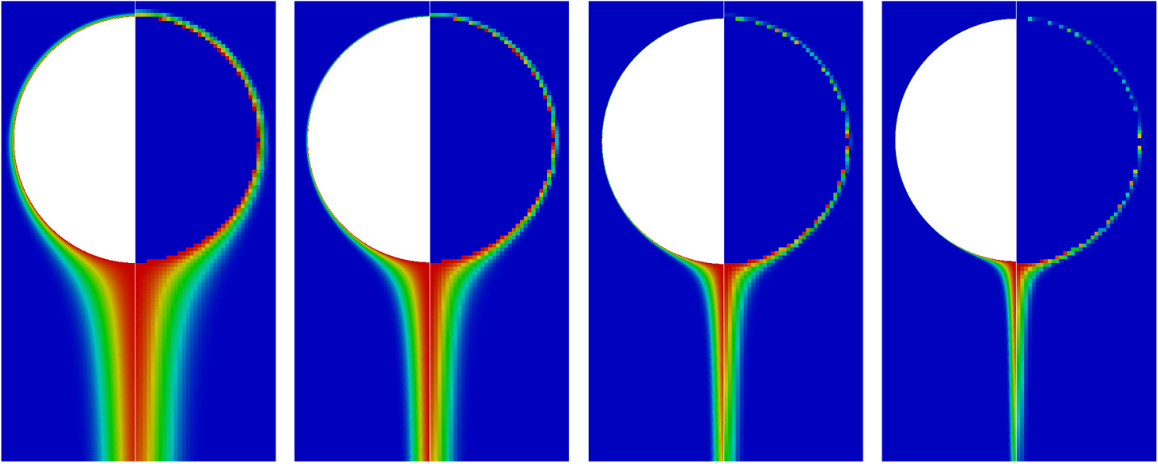Normalized concentration field of Stokes-flow reference solution (left half) and subgrid-scale solution (right half) for $Sc=\nu/D=10^4/10^5/10^6/10^7$ (left to right).

## Results

$Sh = \frac{k_L d_b}{D}$   with   $k_L = \frac{\dot{N}}{A_{eff}\Delta c}$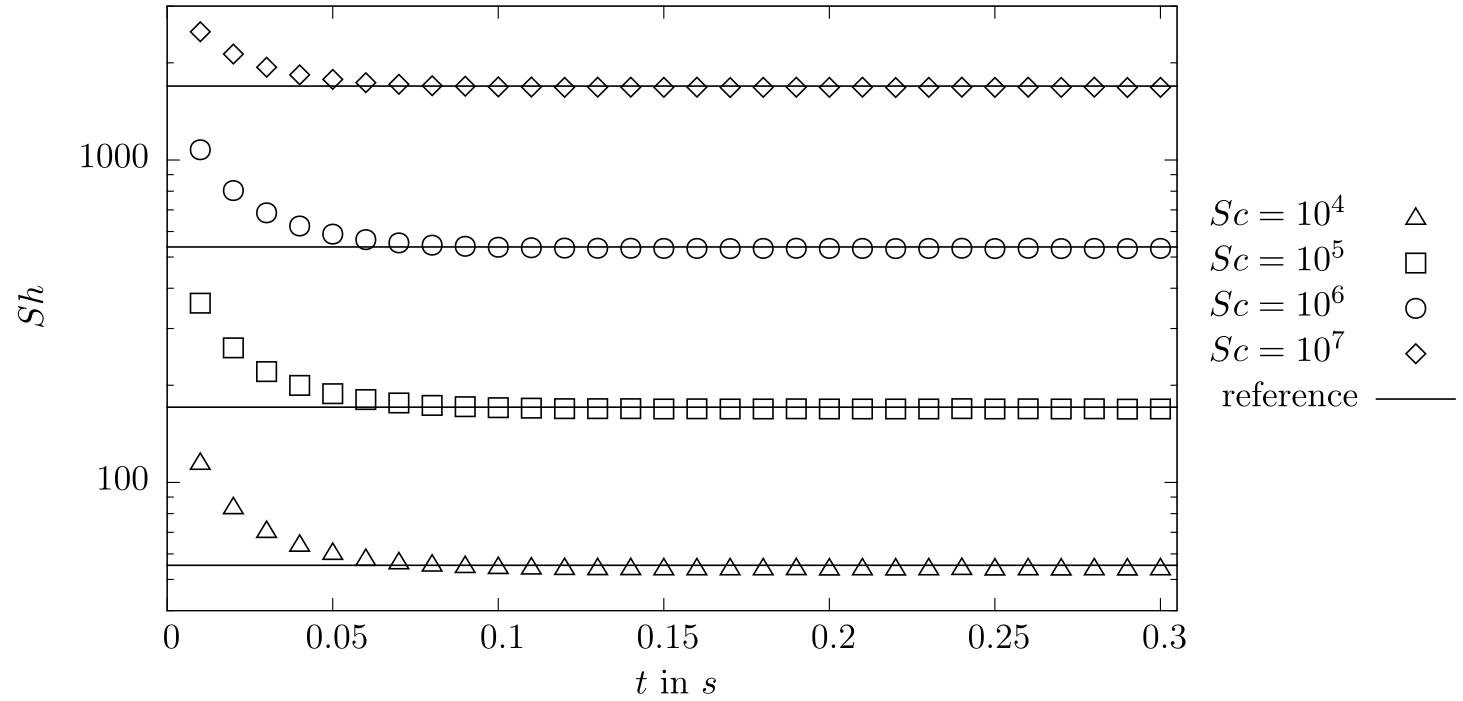## Data-driven modeling

Idea: Replace analytical solution with machine learning (ML) model.

1. Substitute problem
Find a representative substitute problem and solve it numerically to obtain boundary layer data.
2. Model creation
Select meaningful features (variables) and train a ML model.
3. Correction
Correct convective fluxes, diffusive fluxes, and reaction source terms based on the ML model.

## ML terminology

$s$ instances of feature - label pairs

$s$ $x_{1,s}$ $x_{2,s}$ $x_{3,s}$ $y_s$
1 0.1 0.4 0.6 0.5
2 ... ... ... ...

ML model
prediction
error norm
$f_m = f_m(x_{1}, x_{2}, x_{3})$
$\hat{y}_s = f_m(x_{1,s}, x_{2,s}, x_{3,s})$
$L_i = L_i(y_s - \hat{y}_s)$

## (1) Substitute problem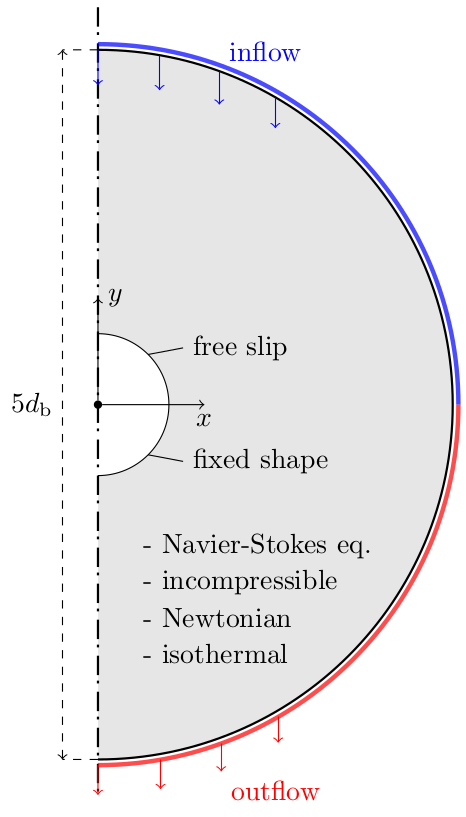numerical solution

• OpenFOAM®
• parameters: $Re$, $Sc_i$, $Da_i$, shape, ...
• finished in minutes

data extraction

• features (input variables) should be available in target simulation
• features motivated by boundary layer theory and previous modeling
• down-sampling to coarse meshes
• stored as comma separated values (csv)

## (2) Model creation

Multilayer Perceptron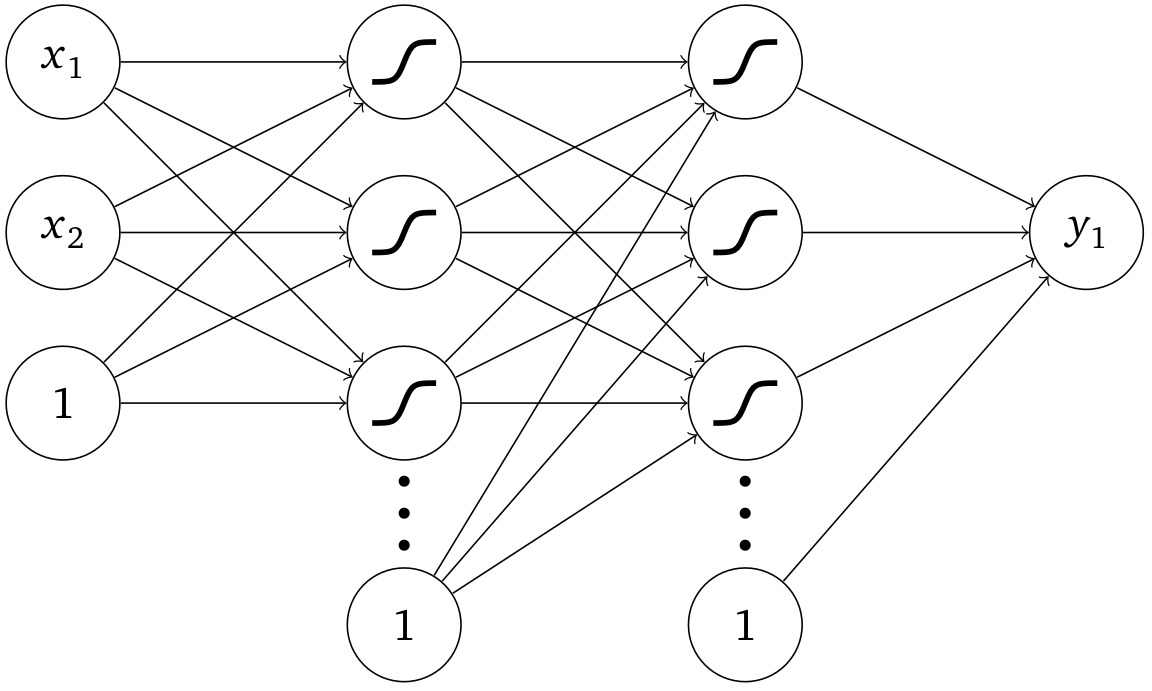• 6 layers with 40 neurons per layer
• SELU activation function

model training

• mean squared error loss
• one model per label
• ≈ 5 min training time per model
• training on CPU with double precision

implementation

• PyTorch®
• export to TorchScript

## SGS Model assessment

Aim:
Create reference data for complex shapes and flow scenarios to assess model generalization.

Idea:
Decoupling of two-phase flow and species transport.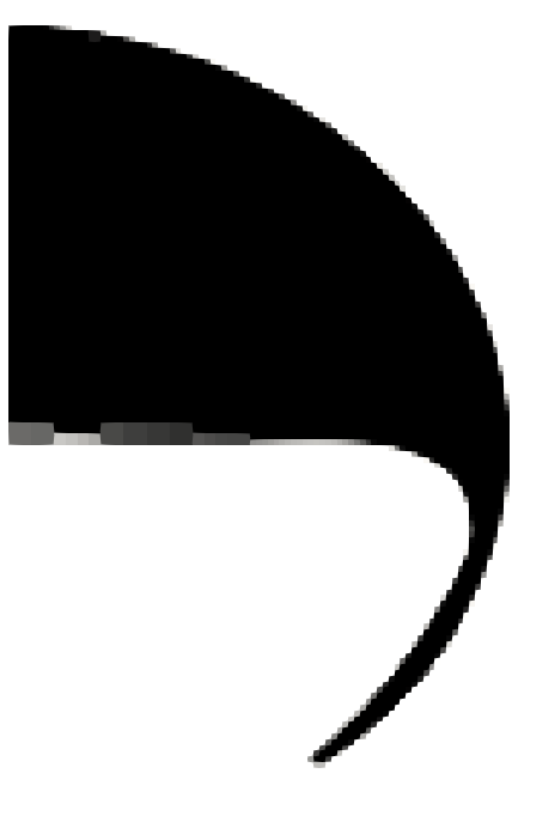1. Two-phase flow simulation (Volume-of-Fluid)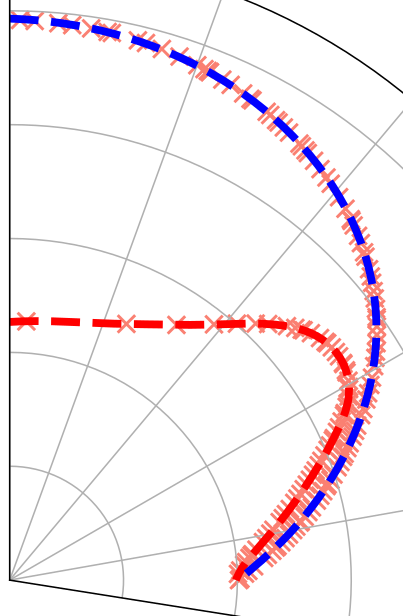2. Parametrization of shape and interfacial velocity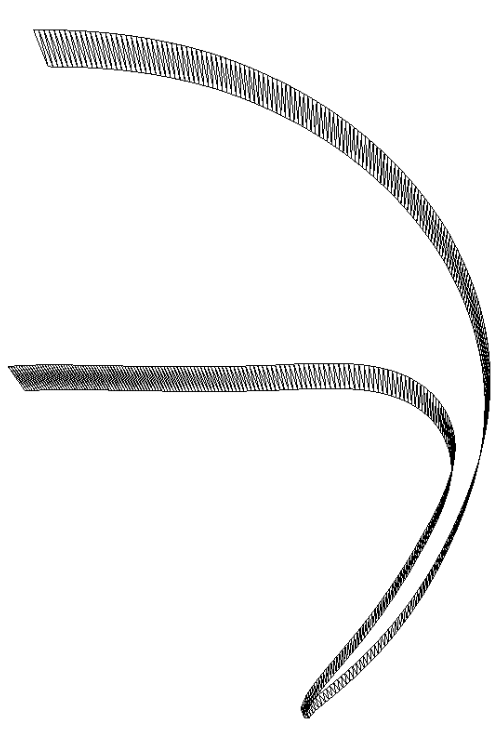3. Geometry generation and export (STL format)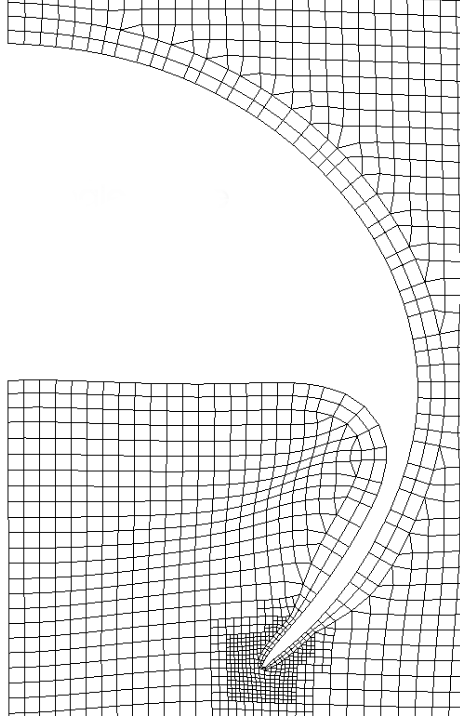4. Single phase mesh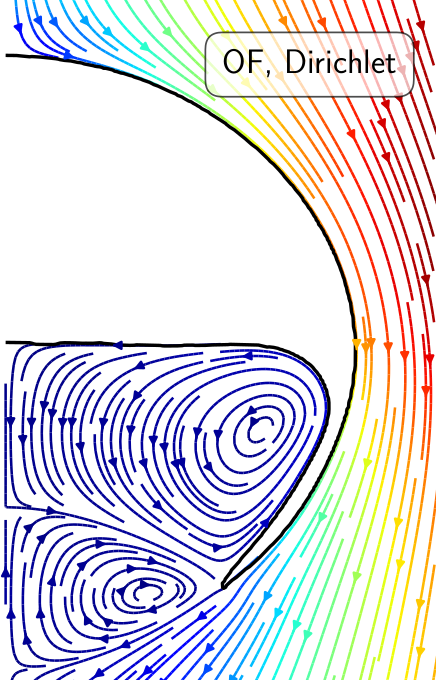5. Flow solution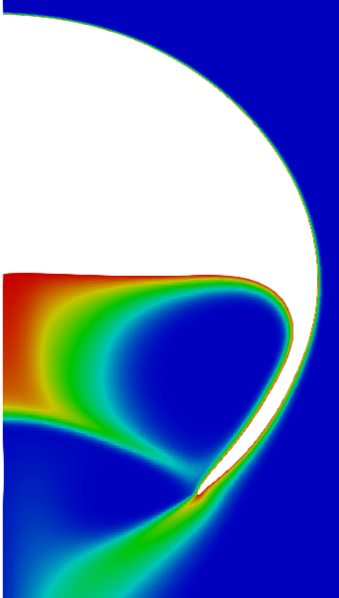6. Species transport

## Mesh dependency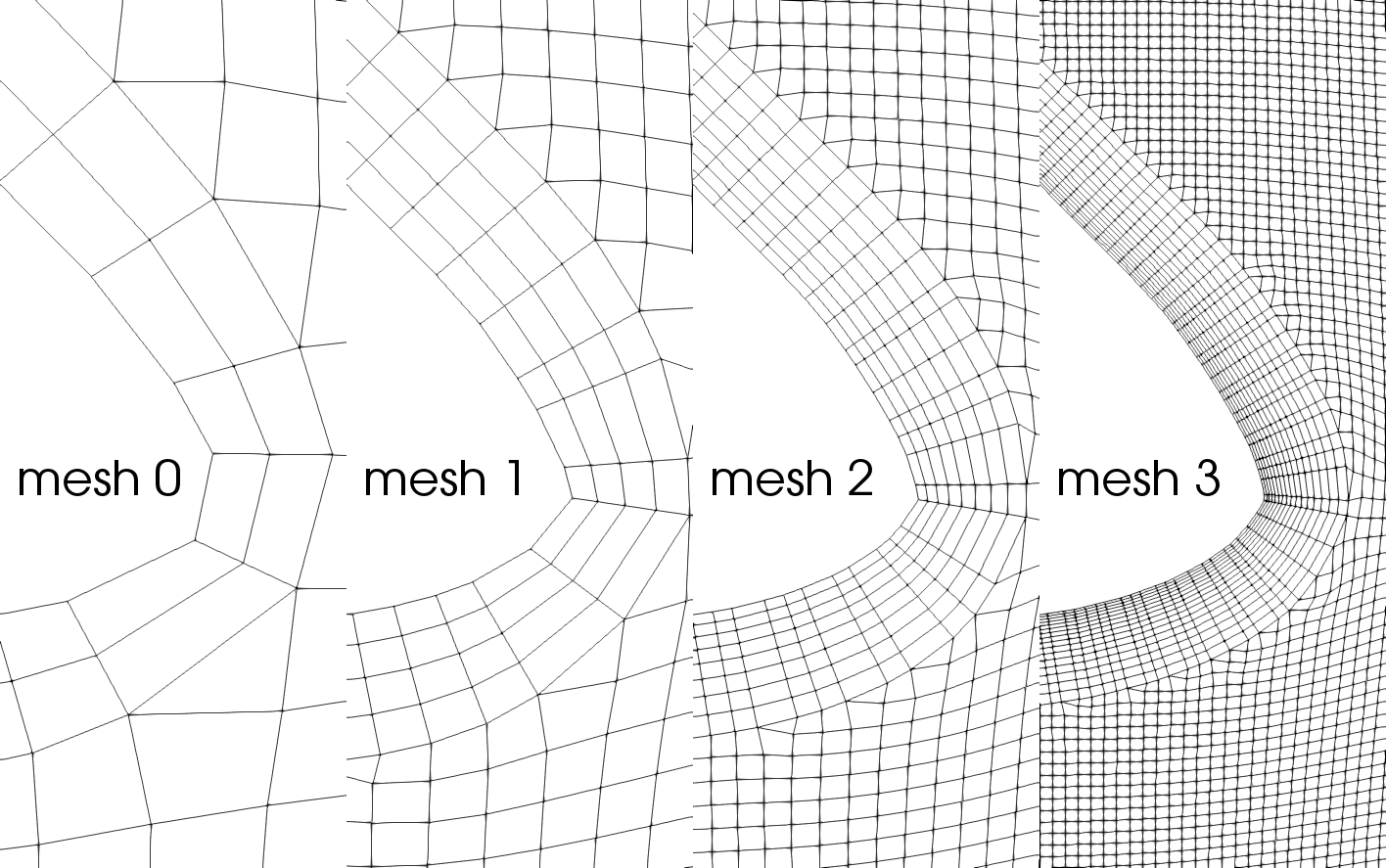Prismatic cell layers around a spherical-cap bubble with increasing mesh resolution.

## Model assessment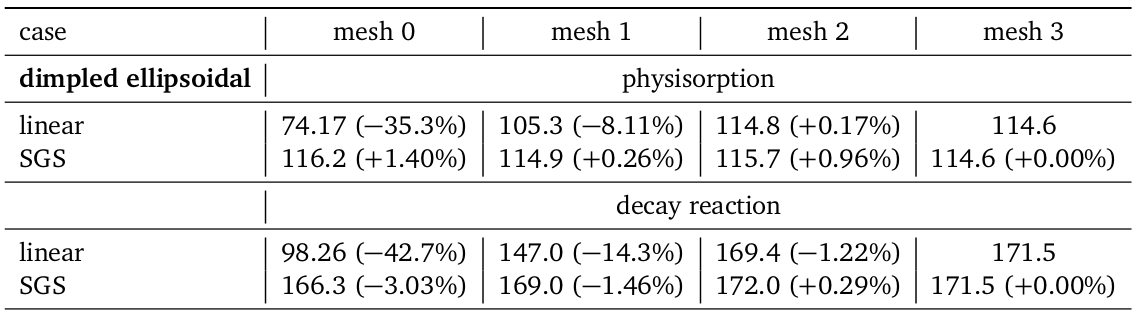Comparison of the global Sherwood number computed with standard discretization (linear) and subgrid-scale model (SGS) on several meshes. The reference value can be found in the rightmost column.

## Comparison with experiments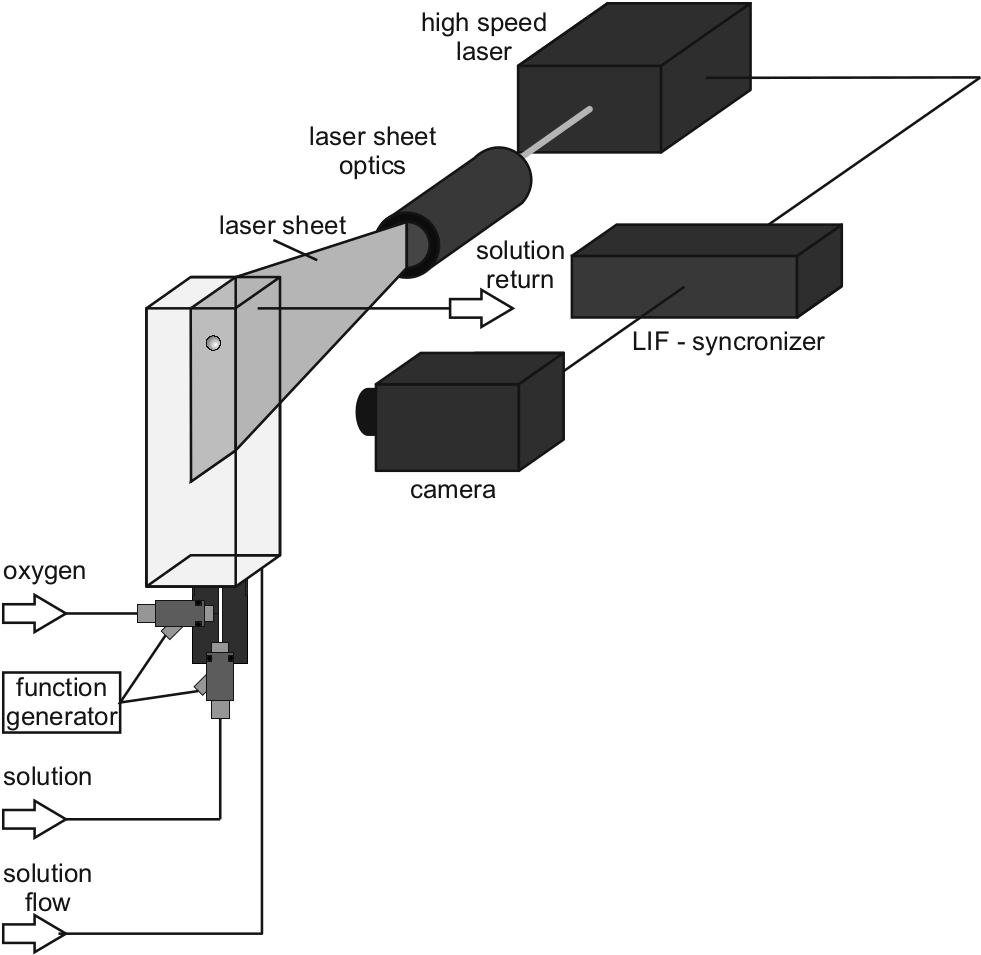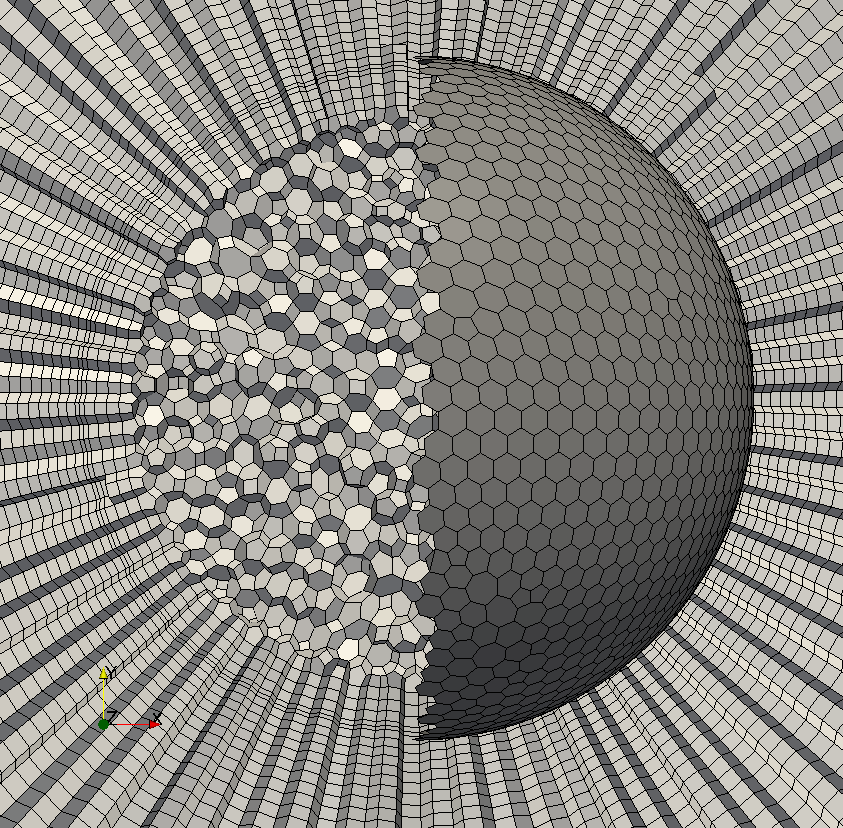Comparsion of the reactive mass transfer at small oxygen bubbles $d_b < 1~mm$ rising in water. Reaction: sulfite-sulfate oxidation. Measurement principle: laser-induced fluorescence.

## Mathematical model

mass balance and jump
$\frac{\partial \rho}{\partial t} + \nabla \cdot \left( \rho\mathbf{u} \right) = 0\quad\text{in}\quad \Omega^\pm (t)$
$[\![ \mathbf{u}] \!] \cdot \mathbf{n}_\Sigma = 0\quad\text{on}\quad \Sigma (t)$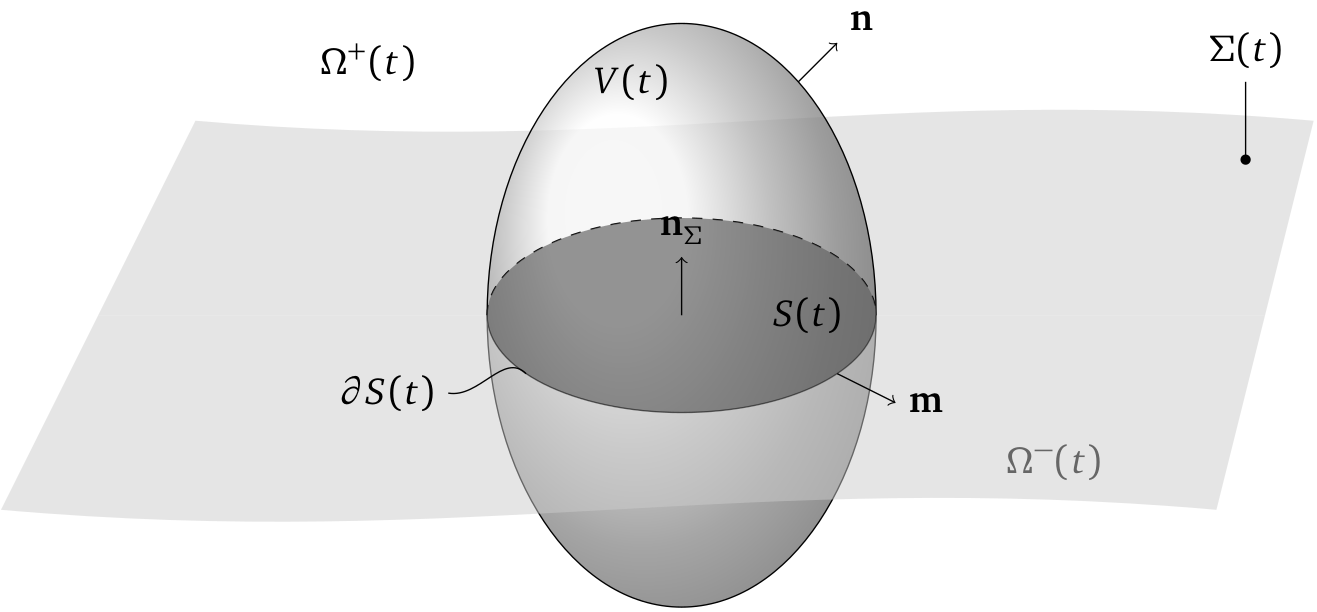momentum balance and jump
$\frac{\partial \rho \mathbf{u}}{\partial t} + \nabla \cdot \left( \rho \mathbf{u} \otimes \mathbf{u} \right) = -\nabla p + \nabla \cdot \left( \eta \left( \nabla \mathbf{u} + \nabla \mathbf{u}^\mathsf{T} \right) \right) + \rho\mathbf{b} \quad\text{in}\quad \Omega^\pm (t)$
$[\![ p\mathbf{I} - \eta \left( \nabla \mathbf{u} + \nabla \mathbf{u}^\mathsf{T} \right)]\!] \cdot \mathbf{n}_\Sigma = \sigma\kappa\mathbf{n}_\Sigma + \nabla_\Sigma \sigma\quad\text{on}\quad \Sigma (t)$

## Interface Tracking

• numerical equivalent of sharp interface model
• surface tension isotherms
$\sigma = \sigma (c^\Sigma)$
• sorption library $s^\Sigma + [\![ -D\nabla c ]\!]\cdot \mathbf{n}_\Sigma = 0$
• here: Langmuir fast sorption model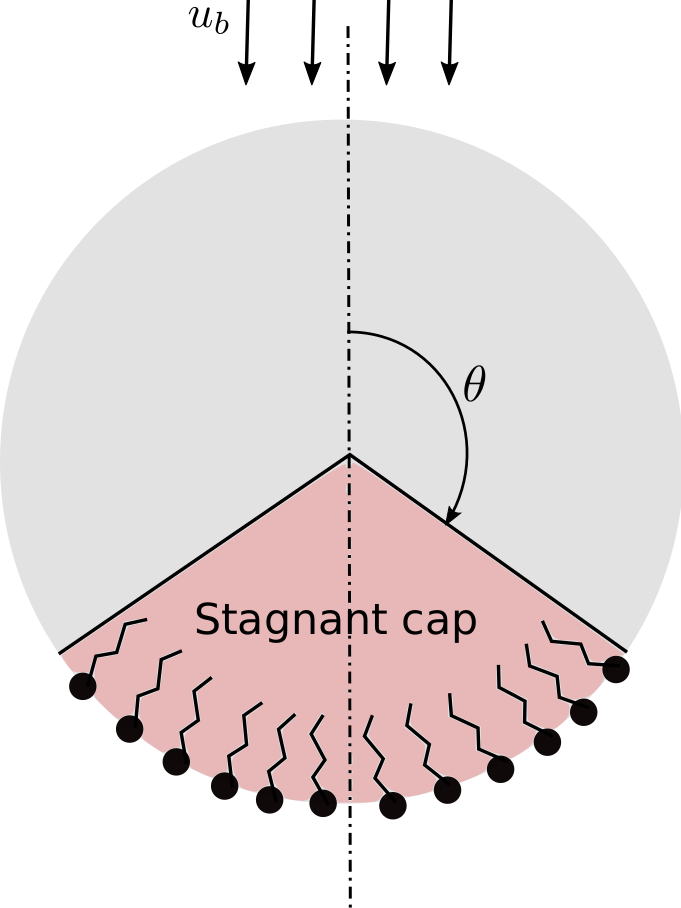Surfactant - surface active agent

## Velocity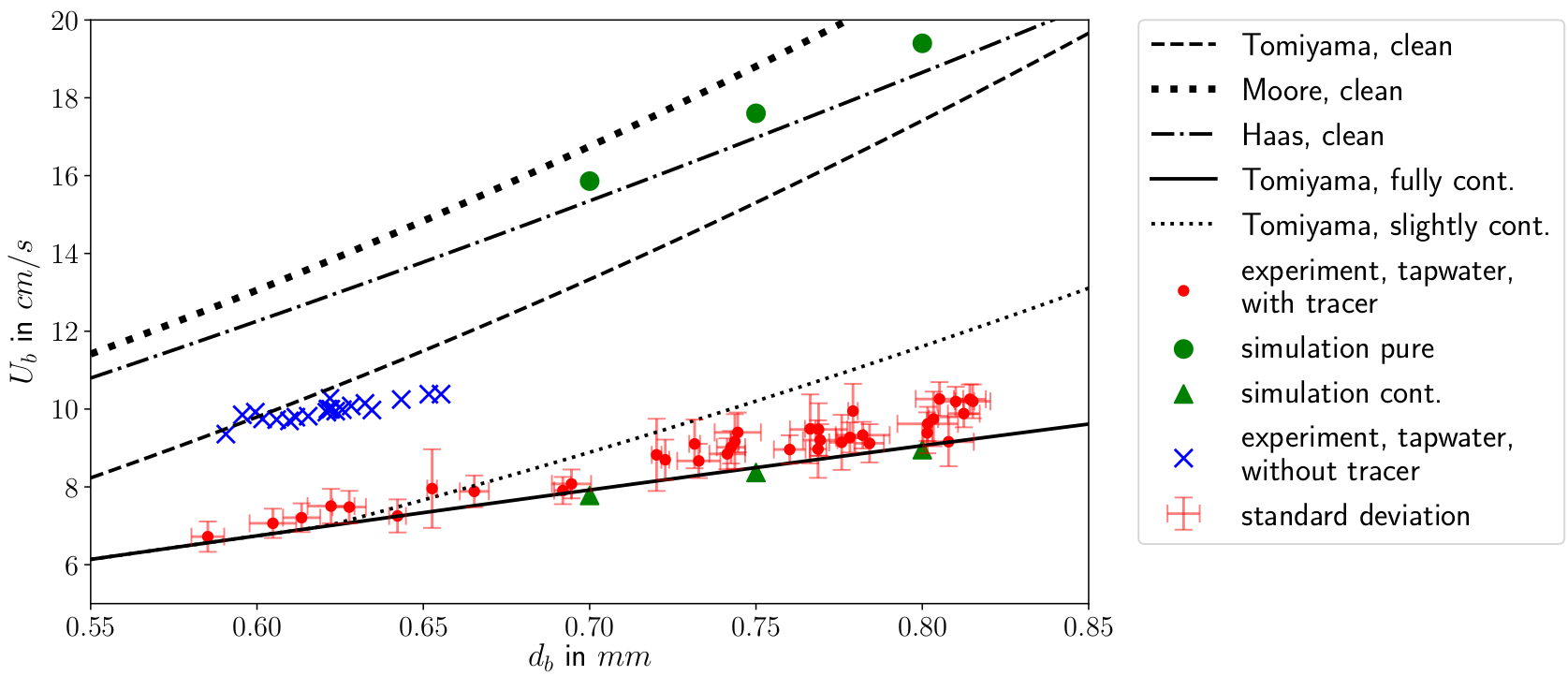Terminal velocity plotted against the bubble diameter. Experimental, numerical, theoretical and literature results.

## Local velocity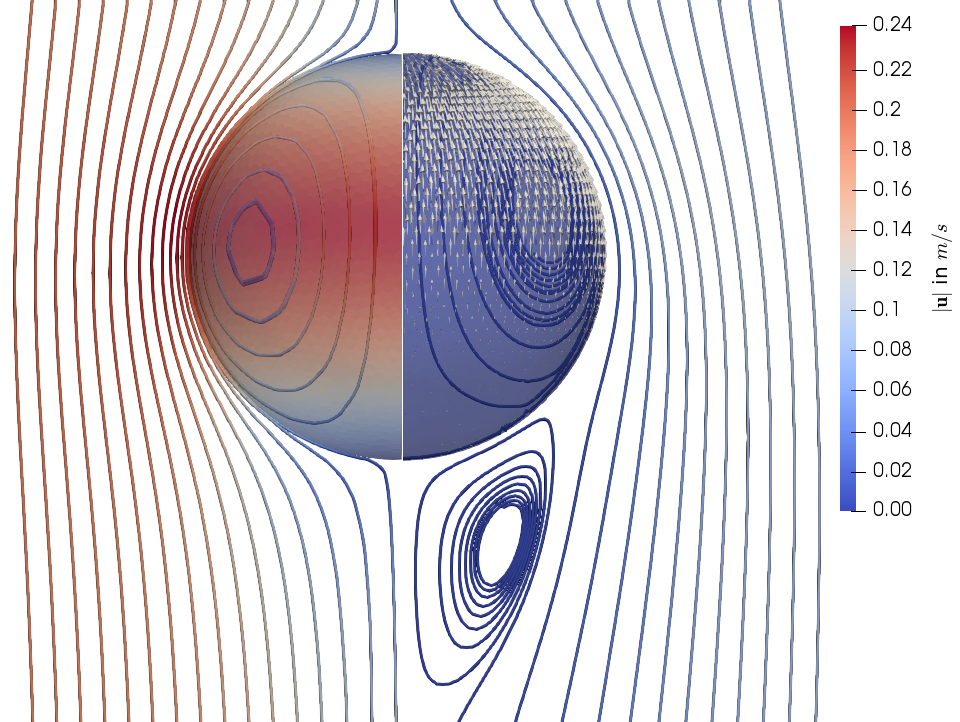Streamlines and magnitude of interfacial velocity for clean (left) and contaminated (right) interfaces. The glyphs depict local Marangoni forces.

## Oxygen transport

Experiment vs. Simulation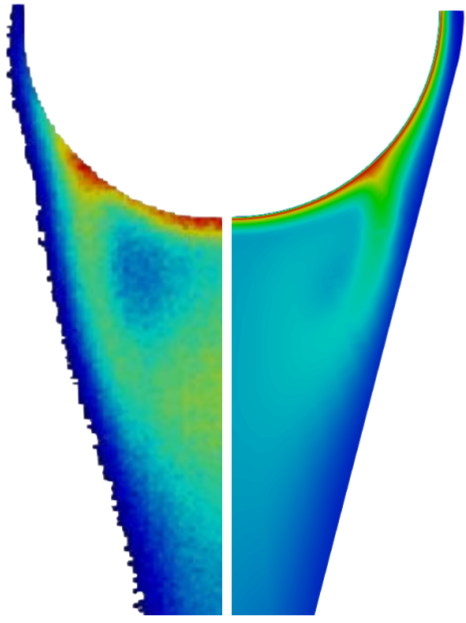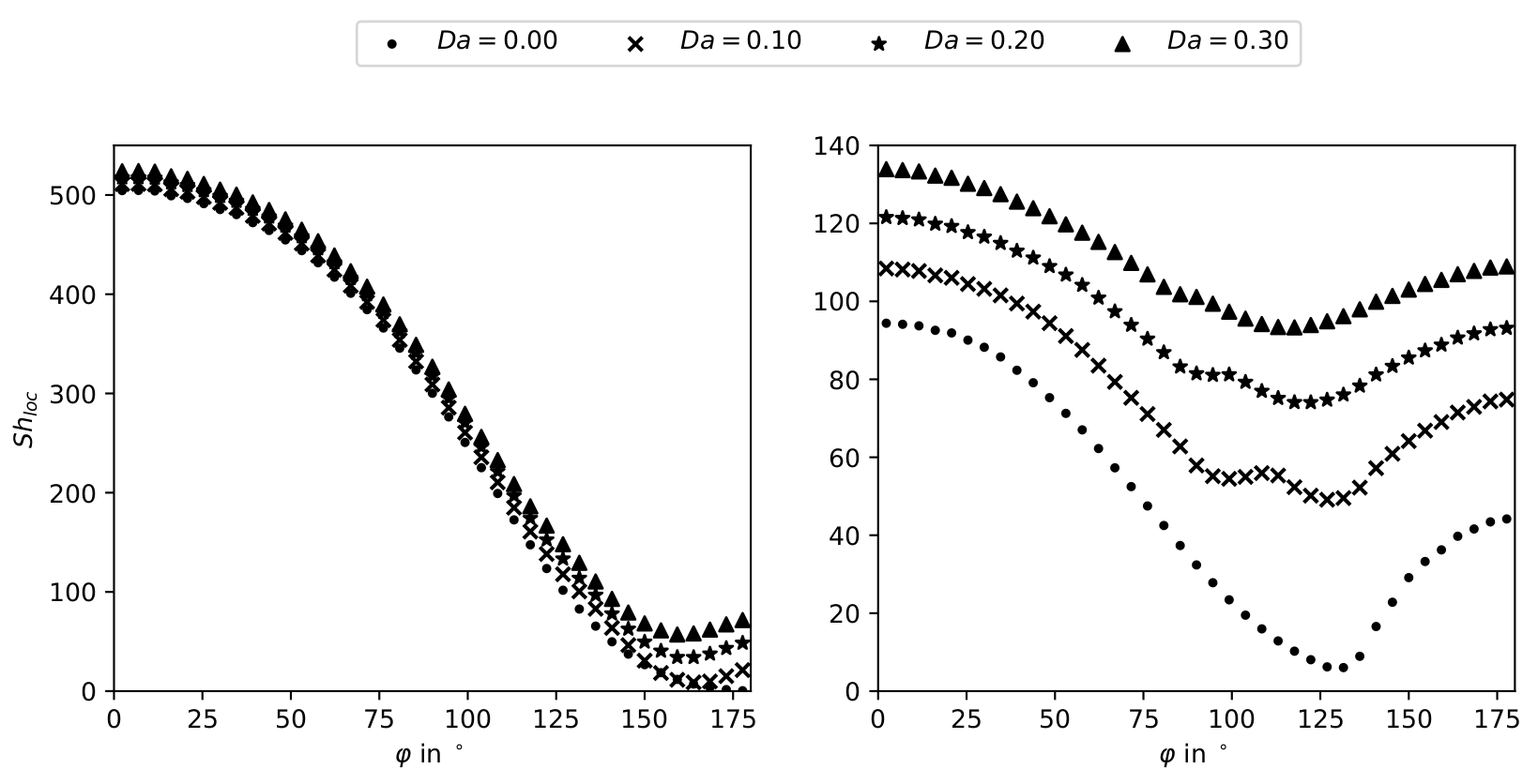Local Sherwood number for clean (left) and contaminated (right) interfaces.
A. Weiner, J. Timmermann, C. Pesci, J. Grewe, M. Hoffmann, M. Schlüter, D. Bothe:
Experimental and numerical investigation of reactive species transport around a small rising bubble (2019)

## Summary

• high-$Pe$ number problem (boundary layer)
• influence on numerical solution
• SGS modeling for complex reactions
• hybrid approach for high-fidelity reference data
• validation with complex bubble shapes
• qualitative and quantitative agreement with experiments

• new understanding of dynamic surfactant adsorption
• well documented, fully reproducible, public research results and methods
• pathway paved for data-driven solutions in computational fluid dynamics

## Outlook

• (data-driven) modeling for the liquid bulk
• assessment for dynamic interfaces
• application to related boundary layer problems

# THE END

Special thanks to David Merker, Jens Timmermann, Chiara Pesci and Dennis Hillenbrand

# Complementary material

## Reproducibility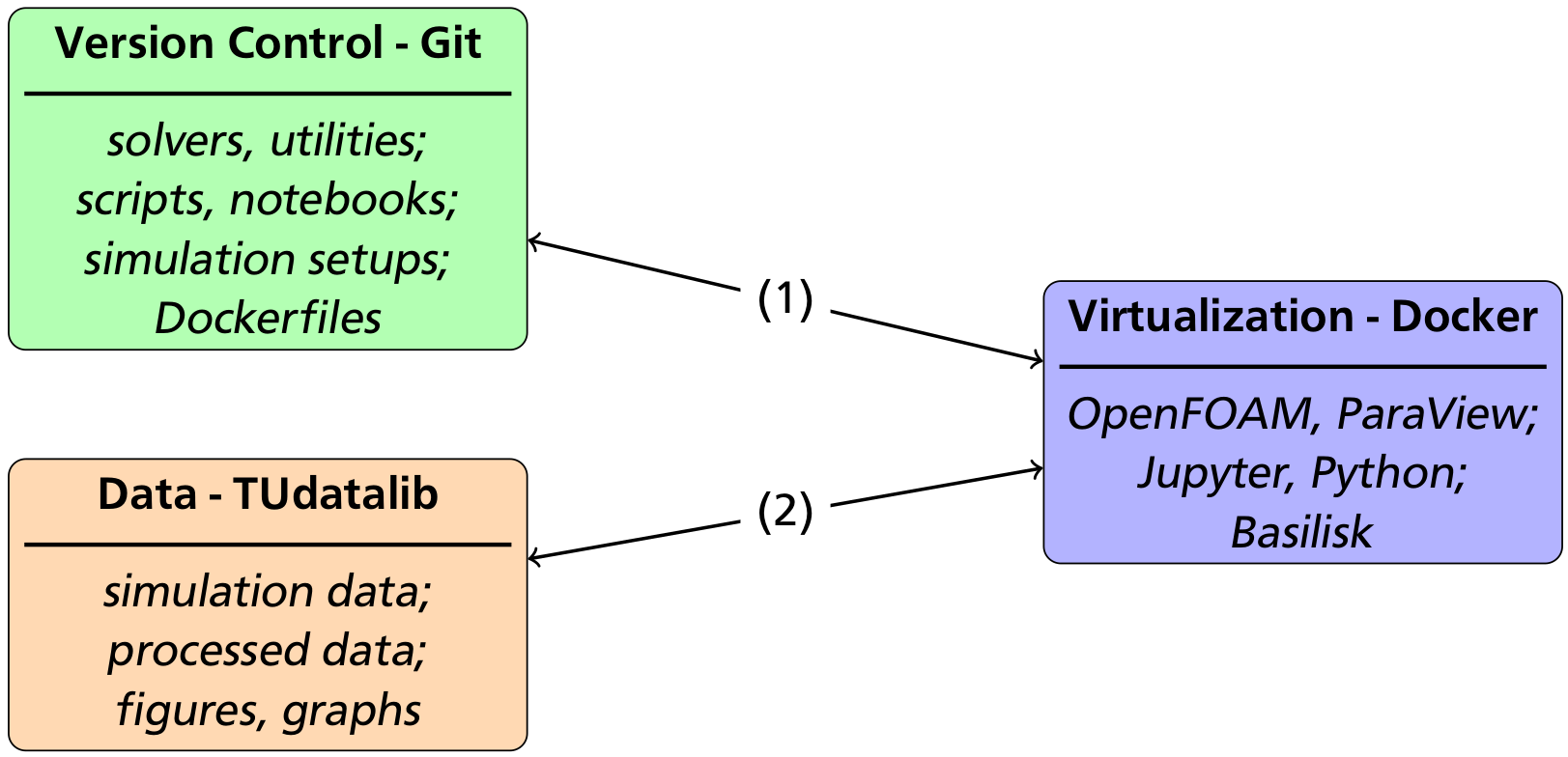Solution based on Git/Github, Docker/Dockerhub and TUDatalib.

## ML Challenges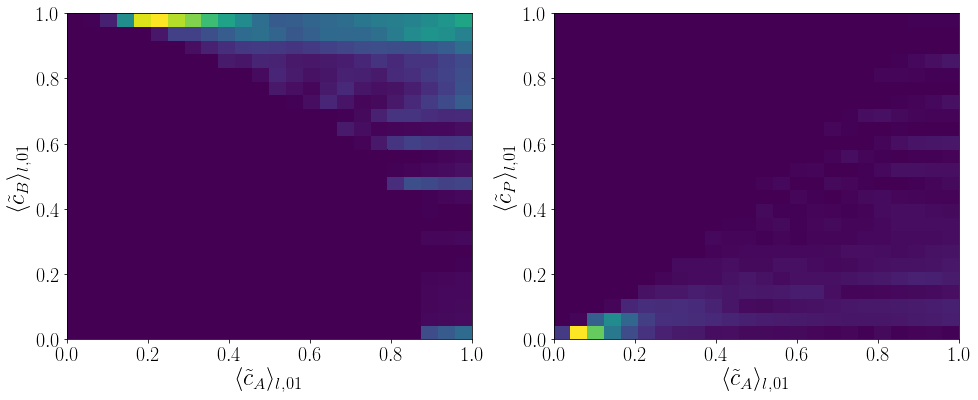Feature density for the source terms of a parallel-consecutive reaction of type $A+B\rightarrow P$ and $A+P\rightarrow S$.

Video by courtesy of David Merker.

## Pendant bubble method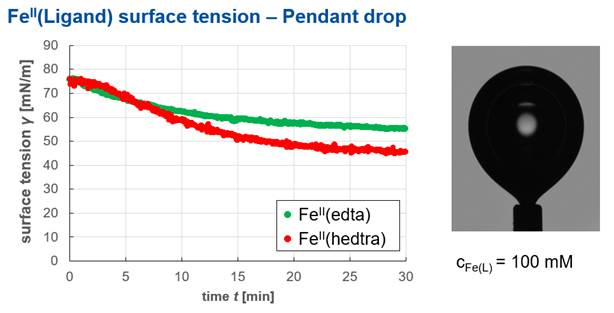Image by courtesy of David Merker. Measurement apparatus by dataphysics-instruments.

## Bulk species - B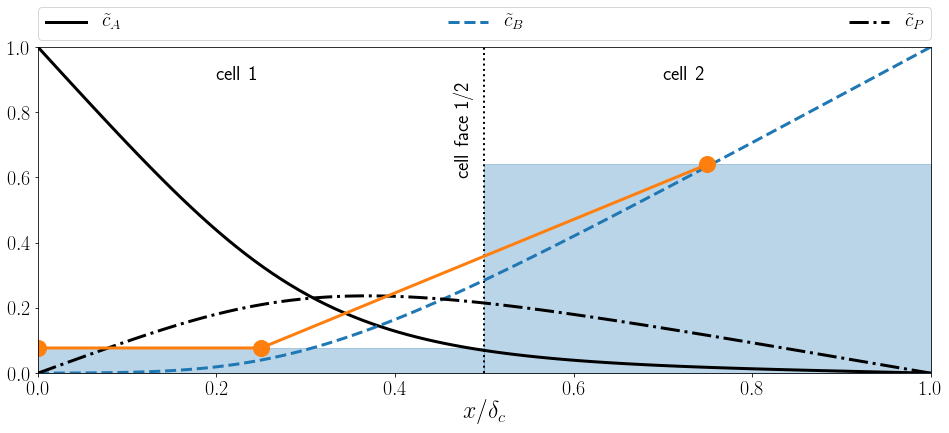Normalized concentration profile $\tilde{c}_B$ in interface normal direction $x/\delta_c$ for the bulk species (dashed blue line), average concentration values per cell (shaded blue), and linear reconstruction (orange).

## Product species - P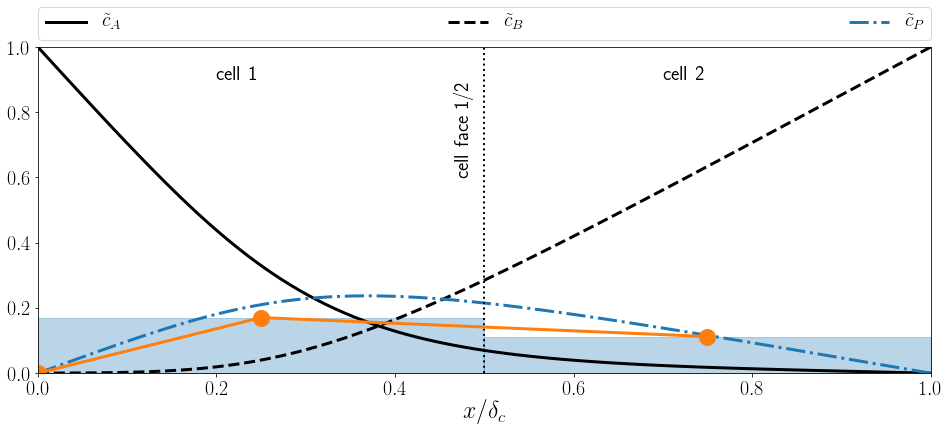Normalized concentration profile $\tilde{c}_P$ in interface normal direction $x/\delta_c$ for the product species (dash-dotted blue line), average concentration values per cell (shaded blue), and linear reconstruction (orange).

## Source term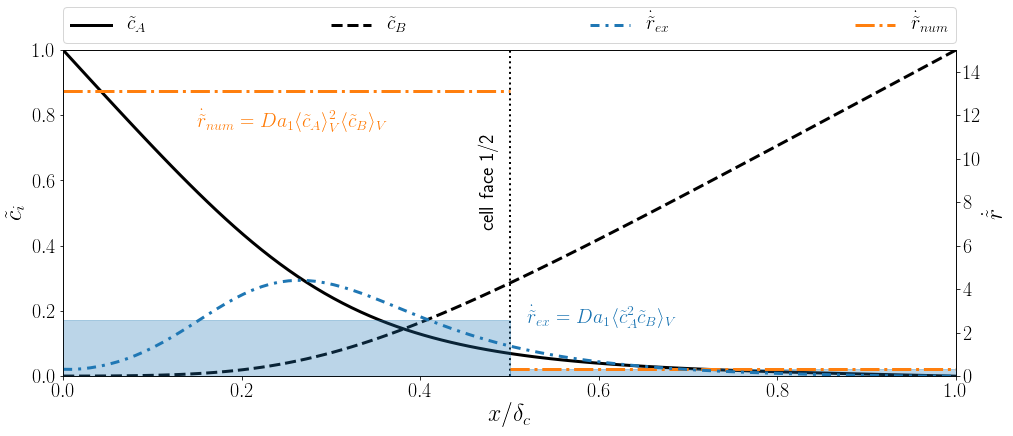Reaction source profile $\dot{\tilde{r}}$ in interface normal direction $x/\delta_c$ (dash-dotted blue line), cell average of $\dot{\tilde{r}}$ (shaded blue), and product of averages (orange). The Damköhler number is defined as $Da=kd_b/U_b$.

## Feature selection

Idea: ranking of feature importance.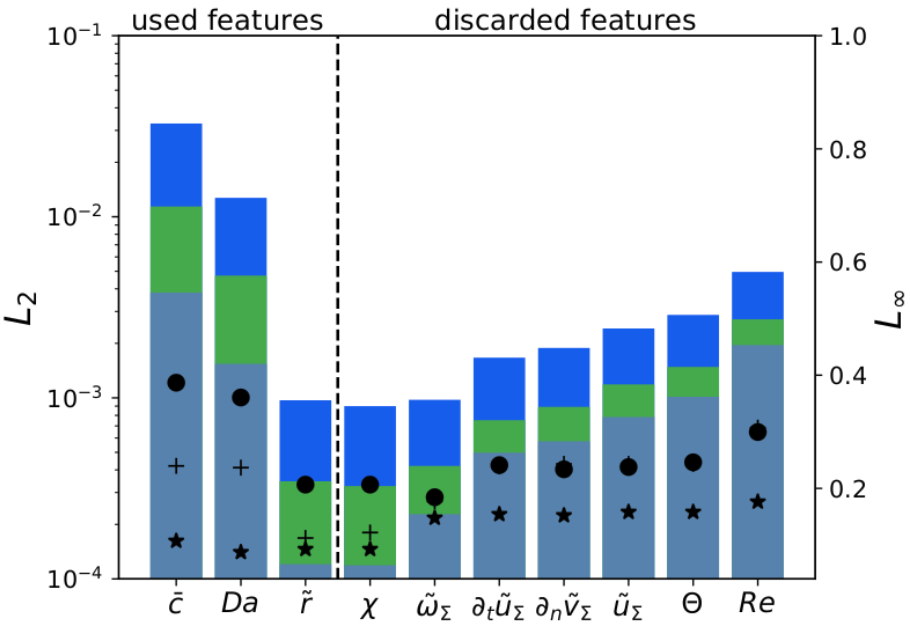algorithms

• regression forest
• sequential backward selection
• sequential forward selection

example (left)

$f_m = f_m(\bar{c},Da,\tilde{r})$

A. Weiner, D. Hillenbrand, H. Marschall, D. Bothe: Data‐Driven Subgrid‐Scale Modeling for Convection‐Dominated Concentration Boundary Layers (2019)

## ML model assessment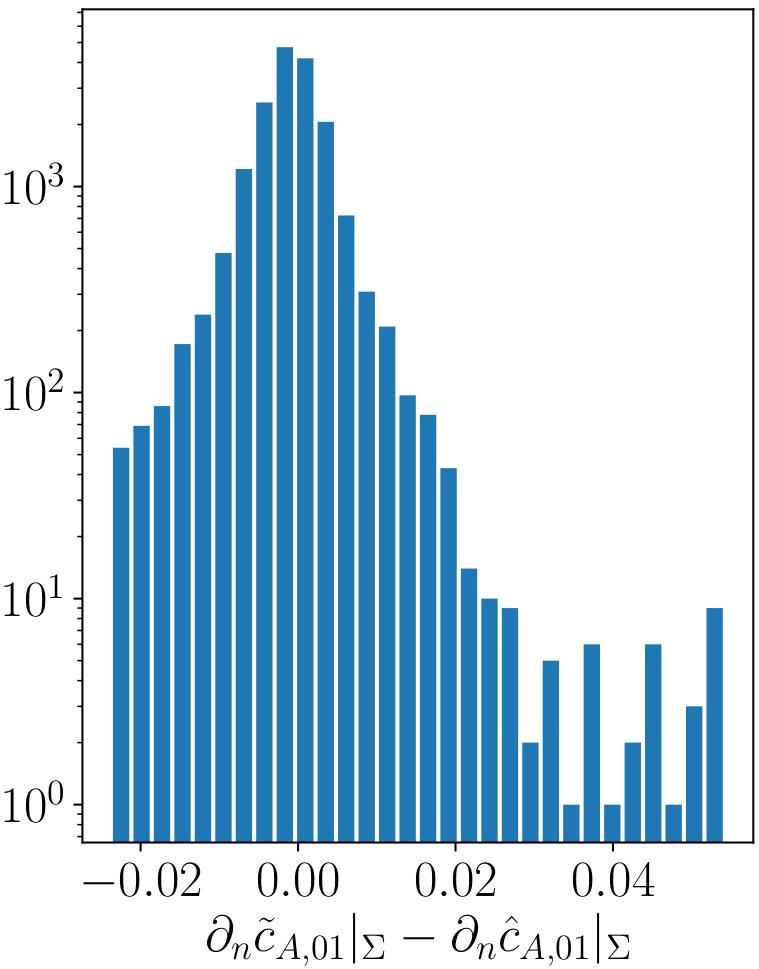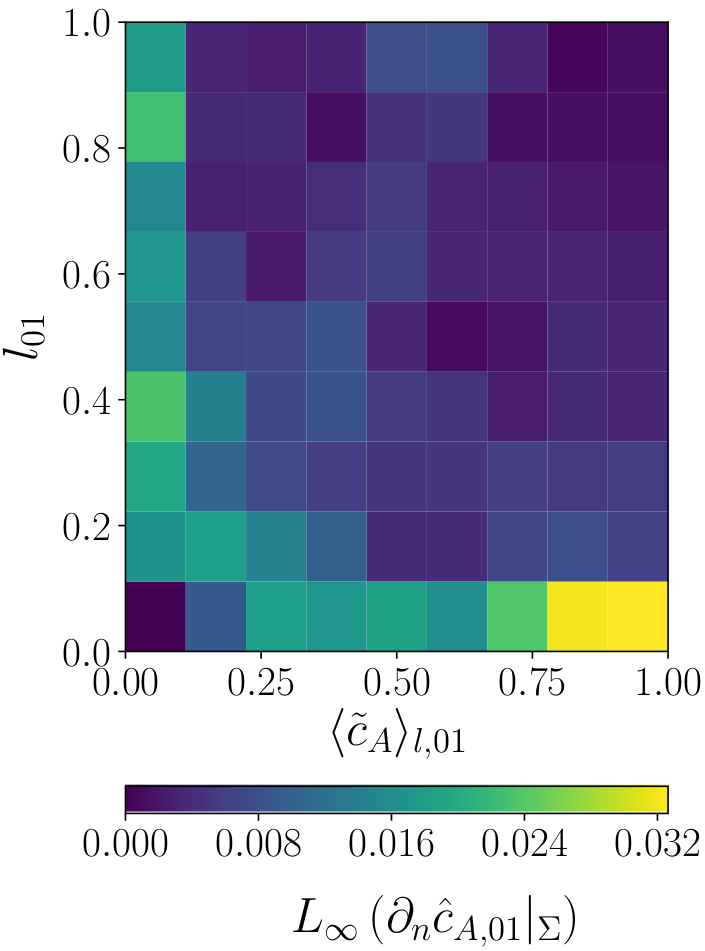Histogram (left) and heatmap (right) of the label error for the transfer species (A). Reminder: the height of a bar in a histogram depicts the number of instances in the range the bar covers. The index $01$ indicates a min-max-scaling.